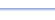• Solves equations of the form
• Finds rational, irrational, and imaginary solutions
• Can be used to factor quadratic polynomials.

Useful for:

• SAT
• ACT
• Algebra 1
• Algebra 2
• Geometry
• Precalculus
• Calculus
• College Algebra

See full list of programs

Example 1

Q: Solve the equation:

Solution:

Enter the values of
A, B, and C:

The program reports:

Interpretation:
x = 2/3 or
x = 1

The third number listed is NOT a solution.  It is the discriminant.  Notice it says 'D=' for this number, not 'X='.  You may want to know the discriminant if you are using the quadratic formula to solve this equation by hand.

See Example 2